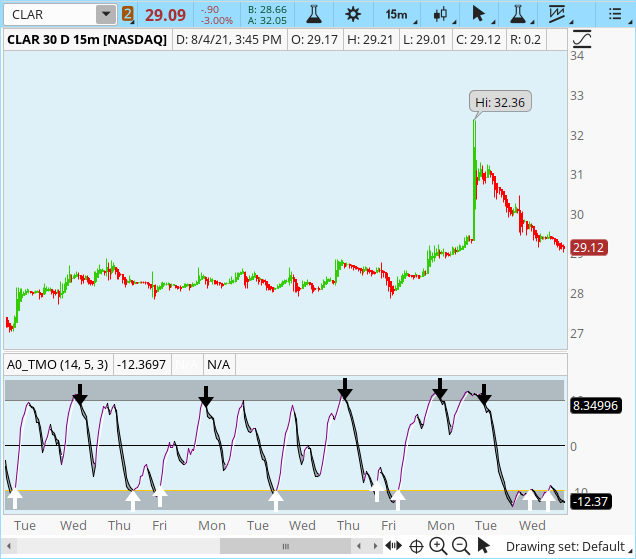# Need help adding long / short arrows to the TMO indicator

#### Noca Jones

##### New member
Hey guys I am trying to add long and short arrows to the TMO indicator that trigger when the main line comes back out of OS or OB. I have tried several times but am having no luck. The code is fine with no errors but the arrows don't paint. I tried to copy the format from other indicators with arrows but still can't get it to work. Here's my code:

Ruby:
``````# TMO ((T)rue (M)omentum (O)scilator)
# Mobius
# V01.05.2018
# hint: TMO calculates momentum using the delta of price. Giving a much better picture of trend, tend reversals and divergence than momentum oscillators using price.

declare Lower;

input length = 14;
input calcLength = 5;
input smoothLength = 3;

def o = open;
def c = close;
def data = fold i = 0 to length
with s
do s + (if c > getValue(o, i)
then 1
else if c < getValue(o, i)
then - 1
else 0);
def EMA5 = ExpAverage(data, calcLength);
plot Main = ExpAverage(EMA5, smoothLength);
plot Signal = ExpAverage(Main, smoothLength);
Main.AssignValueColor(if Main > Signal
then color.white
else color.black);
Signal.AssignValueColor(if Main > Signal
then color.white
else color.black);
Signal.HideBubble();
Signal.HideTitle();
plot zero = if isNaN(c) then double.nan else 0;
zero.SetDefaultColor(Color.black);
zero.hideBubble();
zero.hideTitle();
plot ob = if isNaN(c) then double.nan else round(length * .7);
ob.SetDefaultColor(Color.gray);
ob.HideBubble();
ob.HideTitle();
plot os = if isNaN(c) then double.nan else -round(length * .7);
os.SetDefaultColor(Color.gray);
os.HideBubble();
os.HideTitle();

def LONG = MAIN > OS AND MAIN < OS ;
PLOT UPARROW = LONG;
UPARROW.SetPaintingStrategy(PaintingStrategy.
BOOLEAN_ARROW_UP);
UPARROW.SetDefaultColor(Color.WHITE);
UPARROW.SetLineWeight(5);

def SHORT = MAIN < OB AND MAIN > OB ;

PLOT DOWNARROW = SHORT;

DOWNARROW.SetPaintingStrategy(PaintingStrategy.BOOLEAN_ARROW_UP);
DOWNARROW.SetDefaultColor(Color.BLACK);
DOWNARROW.SetLineWeight(5);

# End Code TMO``````

Here's a screenshot of what I am trying to get:

Last edited by a moderator:

#### MerryDay

Staff member
Staff
VIP
@Noca Jones
TOS requires that you state WHERE on the candle you want the arrow to print. IE: plot it on the main plot, on the signal, on zero, etc... So the statement you need to make is if long is true then print candle at main else print nothing (double.NaN) and you didn't need the boolean logic on your arrows.
So syntax looks like:
Code:
``````def LONG = main crosses above OS;
PLOT UPARROW = if LONG then main else double.NaN ;
UPARROW.SetPaintingStrategy(PaintingStrategy.ARROW_UP);
UPARROW.SetDefaultColor(Color.WHITE);
UPARROW.SetLineWeight(5);

def SHORT = MAIN crosses below OB ;

PLOT DOWNARROW = if SHORT then main else double.NaN ;

DOWNARROW.SetPaintingStrategy(PaintingStrategy.ARROW_down);
DOWNARROW.SetDefaultColor(Color.BLACK);
DOWNARROW.SetLineWeight(5);``````

Sorry for the mix-up before.#### halcyonguy

##### Well-known member
VIP
Hey guys I am trying to add long and short arrows to the TMO indicator that trigger when the main line comes back out of OS or OB. I have tried several times but am having no luck. The code is fine with no errors but the arrows don't paint. I tried to copy the format from other indicators with arrows but still can't get it to work. Here's my code:

# TMO ((T)rue (M)omentum (O)scilator)
# Mobius
# V01.05.2018
# hint: TMO calculates momentum using the delta of price. Giving a much better picture of trend, tend reversals and divergence than momentum oscillators using price.

declare Lower;

input length = 14;
input calcLength = 5;
input smoothLength = 3;

def o = open;
def c = close;
def data = fold i = 0 to length
with s
do s + (if c > getValue(o, i)
then 1
else if c < getValue(o, i)
then - 1
else 0);
def EMA5 = ExpAverage(data, calcLength);
plot Main = ExpAverage(EMA5, smoothLength);
plot Signal = ExpAverage(Main, smoothLength);
Main.AssignValueColor(if Main > Signal
then color.white
else color.black);
Signal.AssignValueColor(if Main > Signal
then color.white
else color.black);
Signal.HideBubble();
Signal.HideTitle();
plot zero = if isNaN(c) then double.nan else 0;
zero.SetDefaultColor(Color.black);
zero.hideBubble();
zero.hideTitle();
plot ob = if isNaN(c) then double.nan else round(length * .7);
ob.SetDefaultColor(Color.gray);
ob.HideBubble();
ob.HideTitle();
plot os = if isNaN(c) then double.nan else -round(length * .7);
os.SetDefaultColor(Color.gray);
os.HideBubble();
os.HideTitle();

def LONG = MAIN > OS AND MAIN < OS ;
PLOT UPARROW = LONG;
UPARROW.SetPaintingStrategy(PaintingStrategy.
BOOLEAN_ARROW_UP);
UPARROW.SetDefaultColor(Color.WHITE);
UPARROW.SetLineWeight(5);

def SHORT = MAIN < OB AND MAIN > OB ;

PLOT DOWNARROW = SHORT;

DOWNARROW.SetPaintingStrategy(PaintingStrategy.BOOLEAN_ARROW_UP);
DOWNARROW.SetDefaultColor(Color.BLACK);
DOWNARROW.SetLineWeight(5);

# End Code TMO

Here's a screenshot of what I am trying to get:

just because there are no errors, doesn't mean the code is ok.

your os and ob formulas are wrong.
you need to fix what is inside of the round( ) functions.
the values will never result in a true condition in LONG = or SHORT =.

length =14
plot ob = if isNaN(c) then double.nan else round(length * .7);
plot os = if isNaN(c) then double.nan else -round(length * .7);

plot Main = ExpAverage(EMA5, smoothLength);

def LONG = MAIN > OS AND MAIN < OS ;
PLOT UPARROW = LONG;

#### Noca Jones

##### New member
@MerryDay Thank you so much. That was the ticket! Working perfectly!

•MerryDay